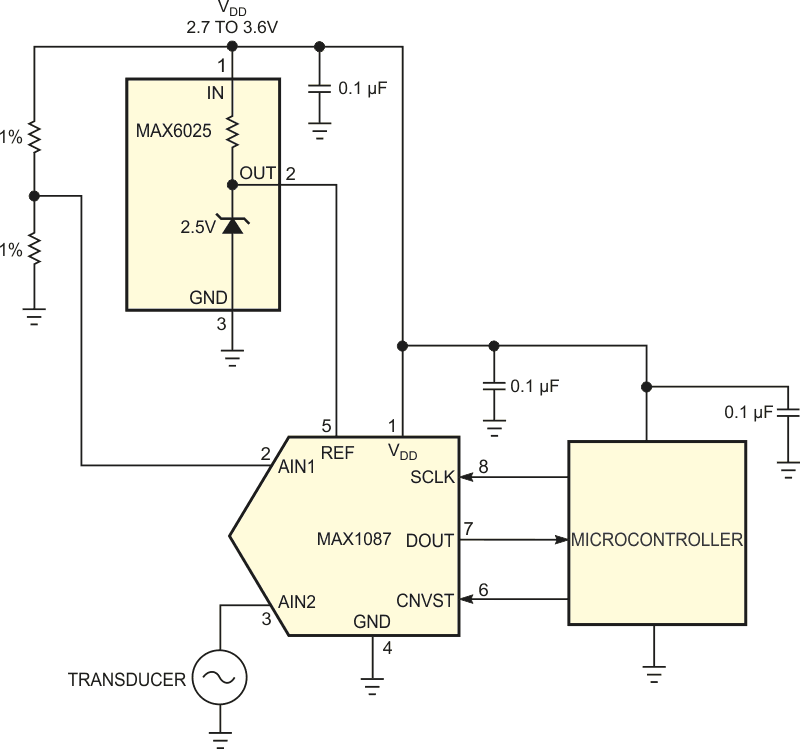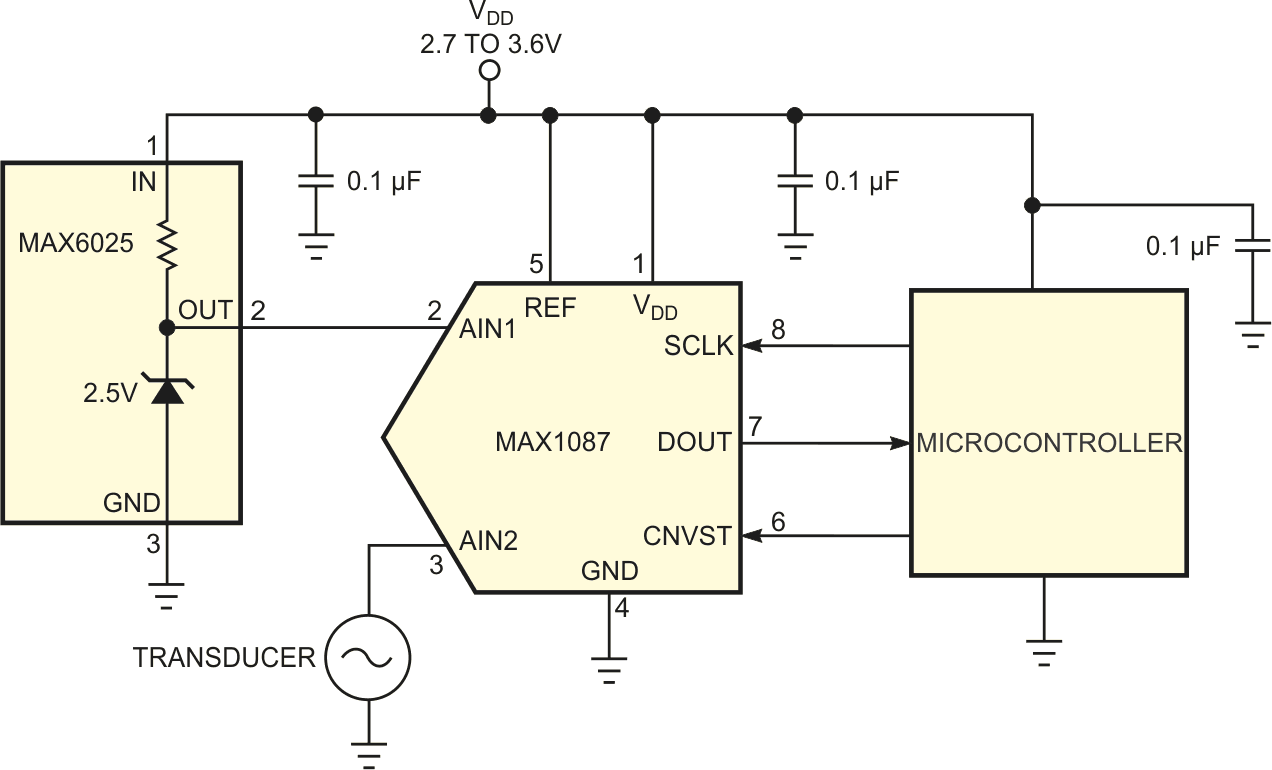# Rearranged reference helps ADC measure its own supply voltage

## Maxim MAX6025 MAX1087

Björn Starmark, Orville Buenaventura, Sören Käck

EDN

If you use an ADC to monitor a system's power-supply voltage, you may encounter situations in which the supply voltage exceeds the ADC's reference voltage (Figure 1). However, the ADC's input voltage cannot exceed its reference voltage. You can use an external resistive divider to bring the supply voltage within the ADC's input range, but even 0.1%-tolerance resistors may introduce an objectionable error. You can solve the problem by eliminating the divider, connecting the ADC's reference input to its power supply, and connecting one of the ADC's inputs to a precision voltage reference – in this case, a 2.5 V MAX6025A (Figure 2).Figure 1. A precision resistive divider brings the power-supply voltage within this ADC’s input range but introduces measurement errors.

In this configuration, the ADC measures its inputs with respect to the supply voltage. Using the digitized reference voltage as a standard, the system's software computes the ratio of the reference voltage with respect to the power-supply voltage and corrects the remaining inputs' measurements. The ADC must accommodate an external reference voltage that equals its power-supply voltage, and any noise on the supply rail disturbs measurements of all channels. Thus, to quiet the supply rail in electrically noisy environments, you may need to add a lowpass filter to provide extra decoupling at the ADC.Figure 2. To eliminate the resistive divider, you connect the ADC’s reference voltage to its power supply and measure the precision voltage reference’s output.

EDN